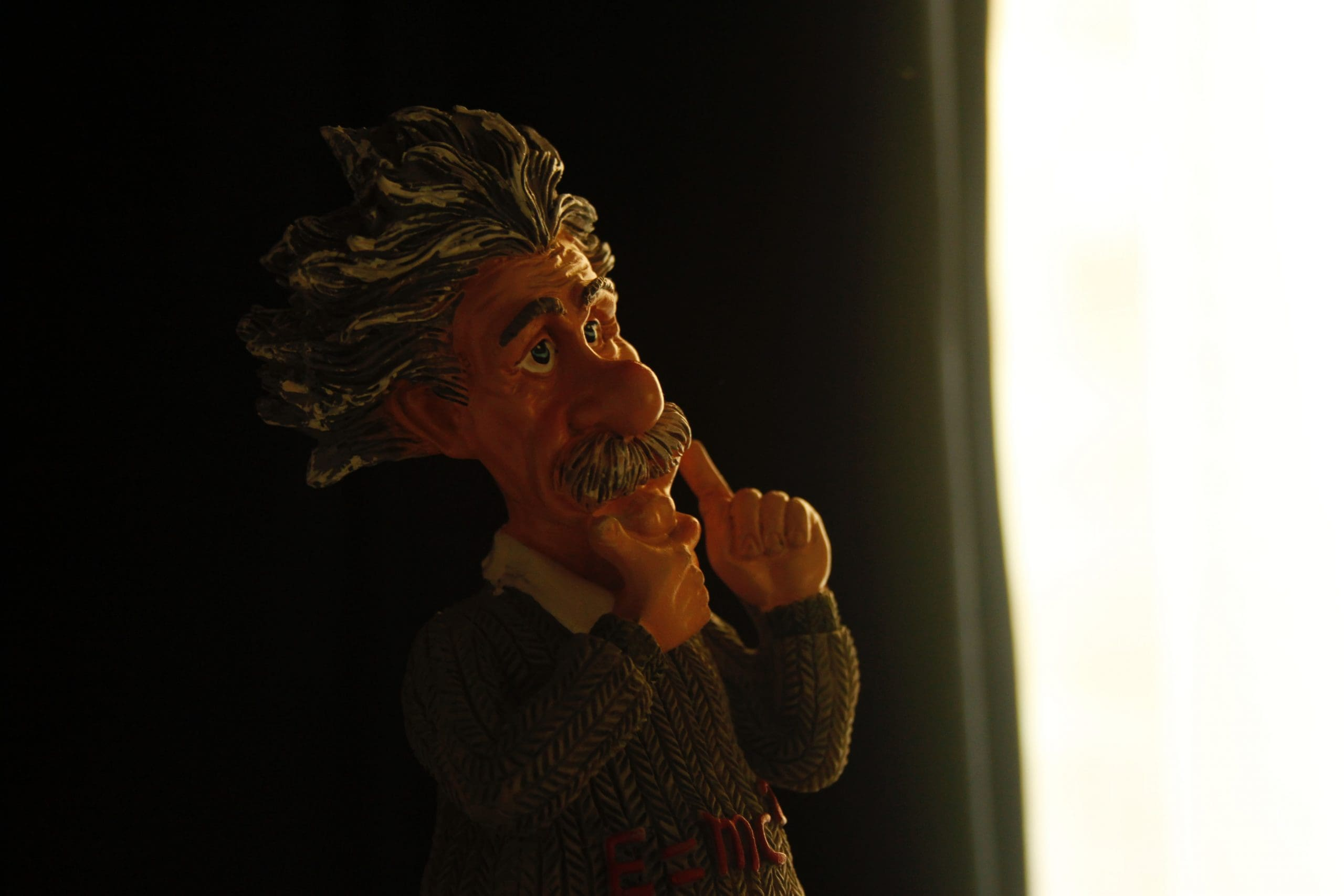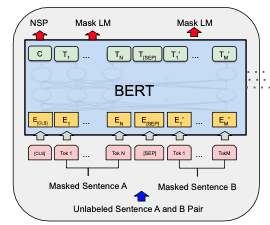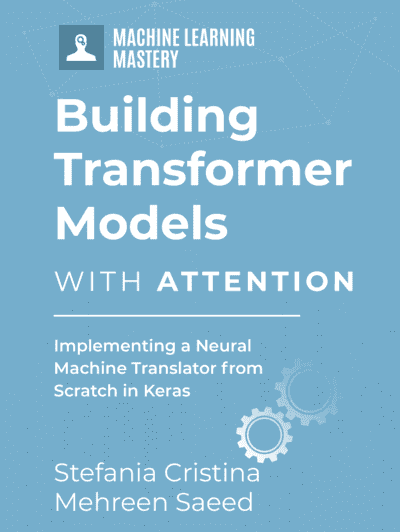# A Brief Introduction to BERT

As we learned what a Transformer is and how we might train the Transformer model, we notice that it is a great tool to make a computer understand human language. However, the Transformer was originally designed as a model to translate one language to another. If we repurpose it for a different task, we would likely need to retrain the whole model from scratch. Given the time it takes to train a Transformer model is enormous, we would like to have a solution that enables us to readily reuse the trained Transformer for many different tasks. BERT is such a model. It is an extension of the encoder part of a Transformer.

In this tutorial, you will learn what BERT is and discover what it can do.

After completing this tutorial, you will know:

• What is a Bidirectional Encoder Representations from Transformer (BERT)
• How a BERT model can be reused for different purposes
• How you can use a pre-trained BERT model

Kick-start your project with my book Building Transformer Models with Attention. It provides self-study tutorials with working code to guide you into building a fully-working transformer model that can
translate sentences from one language to another...

Let’s get started.A brief introduction to BERT
Photo by Samet Erköseoğlu, some rights reserved.

## Tutorial Overview

This tutorial is divided into four parts; they are:

• From Transformer Model to BERT
• What Can BERT Do?
• Using Pre-Trained BERT Model for Summarization
• Using Pre-Trained BERT Model for Question-Answering

## Prerequisites

For this tutorial, we assume that you are already familiar with:

## From Transformer Model to BERT

In the transformer model, the encoder and decoder are connected to make a seq2seq model in order for you to perform a translation, such as from English to German, as you saw before. Recall that the attention equation says:

$$\text{attention}(Q,K,V) = \text{softmax}\Big(\frac{QK^\top}{\sqrt{d_k}}\Big)V$$

But each of the $Q$, $K$, and $V$ above is an embedding vector transformed by a weight matrix in the transformer model. Training a transformer model means finding these weight matrices. Once the weight matrices are learned, the transformer becomes a language model, which means it represents a way to understand the language that you used to train it.

A transformer has encoder and decoder parts. As the name implies, the encoder transforms sentences and paragraphs into an internal format (a numerical matrix) that understands the context, whereas the decoder does the reverse. Combining the encoder and decoder allows a transformer to perform seq2seq tasks, such as translation. If you take out the encoder part of the transformer, it can tell you something about the context, which can do something interesting.

The Bidirectional Encoder Representation from Transformer (BERT) leverages the attention model to get a deeper understanding of the language context. BERT is a stack of many encoder blocks. The input text is separated into tokens as in the transformer model, and each token will be transformed into a vector at the output of BERT.

## What Can BERT Do?

A BERT model is trained using the masked language model (MLM) and next sentence prediction (NSP) simultaneously.BERT model

Each training sample for BERT is a pair of sentences from a document. The two sentences can be consecutive in the document or not. There will be a [CLS] token prepended to the first sentence (to represent the class) and a [SEP] token appended to each sentence (as a separator). Then, the two sentences will be concatenated as a sequence of tokens to become a training sample. A small percentage of the tokens in the training sample is masked with a special token [MASK] or replaced with a random token.

Before it is fed into the BERT model, the tokens in the training sample will be transformed into embedding vectors, with the positional encodings added, and particular to BERT, with segment embeddings added as well to mark whether the token is from the first or the second sentence.

Each input token to the BERT model will produce one output vector. In a well-trained BERT model, we expect:

• output corresponding to the masked token can reveal what the original token was
• output corresponding to the [CLS] token at the beginning can reveal whether the two sentences are consecutive in the document

Then, the weights trained in the BERT model can understand the language context well.

Once you have such a BERT model, you can use it for many downstream tasks. For example, by adding an appropriate classification layer on top of an encoder and feeding in only one sentence to the model instead of a pair, you can take the class token [CLS] as input for sentiment classification. It works because the output of the class token is trained to aggregate the attention for the entire input.

Another example is to take a question as the first sentence and the text (e.g., a paragraph) as the second sentence, then the output token from the second sentence can mark the position where the answer to the question rested. It works because the output of each token reveals some information about that token in the context of the entire input.

## Using Pre-Trained BERT Model for Summarization

A transformer model takes a long time to train from scratch. The BERT model would take even longer. But the purpose of BERT is to create one model that can be reused for many different tasks.

There are pre-trained BERT models that you can use readily. In the following, you will see a few use cases. The text used in the following example is from:

Theoretically, a BERT model is an encoder that maps each input token to an output vector, which can be extended to an infinite length sequence of tokens. In practice, there are limitations imposed in the implementation of other components that limit the input size. Mostly, a few hundred tokens should work, as not every implementation can take thousands of tokens in one shot. You can save the entire article in article.txt (a copy is available here). In case your model needs a smaller text, you can use only a few paragraphs from it.

First, let’s explore the task for summarization. Using BERT, the idea is to extract a few sentences from the original text that represent the entire text. You can see this task is similar to next sentence prediction, in which if given a sentence and the text, you want to classify if they are related.

To do that, you need to use the Python module bert-extractive-summarizer

It is a wrapper to some Hugging Face models to provide the summarization task pipeline. Hugging Face is a platform that allows you to publish machine learning models, mainly on NLP tasks.

Once you have installed bert-extractive-summarizer, producing a summary is just a few lines of code:

This gives the output:

That’s the complete code! Behind the scene, spaCy was used on some preprocessing, and Hugging Face was used to launch the model. The model used was named distilbert-base-uncased. DistilBERT is a simplified BERT model that can run faster and use less memory. The model is an “uncased” one, which means the uppercase or lowercase in the input text is considered the same once it is transformed into embedding vectors.

The output from the summarizer model is a string. As you specified num_sentences=3 in invoking the model, the summary is three selected sentences from the text. This approach is called the extractive summary. The alternative is an abstractive summary, in which the summary is generated rather than extracted from the text. This would need a different model than BERT.

### Want to Get Started With Building Transformer Models with Attention?

Take my free 12-day email crash course now (with sample code).

Click to sign-up and also get a free PDF Ebook version of the course.

## Using Pre-Trained BERT Model for Question-Answering

The other example of using BERT is to match questions to answers. You will give both the question and the text to the model and look for the output of the beginning and the end of the answer from the text.

A quick example would be just a few lines of code as follows, reusing the same example text as in the previous example:

Here, Hugging Face is used directly. If you have installed the module used in the previous example, the Hugging Face Python module is a dependence that you already installed. Otherwise, you may need to install it with pip:

And to actually use a Hugging Face model, you should have both PyTorch and TensorFlow installed as well:

The output of the code above is a Python dictionary, as follows:

This is where you can find the answer (which is a sentence from the input text), as well as the begin and end position in the token order where this answer was from. The score can be regarded as the confidence score from the model that the answer could fit the question.

Behind the scenes, what the model did was generate a probability score for the best beginning in the text that answers the question, as well as the text for the best ending. Then the answer is extracted by finding the location of the highest probabilities.

This section provides more resources on the topic if you are looking to go deeper.

## Summary

In this tutorial, you discovered what BERT is and how to use a pre-trained BERT model.

Specifically, you learned:

• How is BERT created as an extension to Transformer models
• How to use pre-trained BERT models for extractive summarization and question answering

## Learn Transformers and Attention!...using transformer models with attention

Discover how in my new Ebook:
Building Transformer Models with Attention

It provides self-study tutorials with working code to guide you into building a fully-working transformer models that can
translate sentences from one language to another...

### One Response to A Brief Introduction to BERT

1.James Yu December 8, 2022 at 1:16 pm #

Thanks for sharing the post. Most of the things in the post make sense to me, and I am specially appreciate the demo. I also got a questions though. If I remember correctly, transformer mechanism perform self attention through operations on QKV vectors decoded from existing text. Attention aggregate as a result of high value of attention(Q, K, V) operation. Then, there is an issue I got:

“For example, by adding an appropriate classification layer on top of an encoder and feeding in only one sentence to the model instead of a pair, you can take the class token [CLS] as input for sentiment classification. It works because the output of the class token is trained to aggregate the attention for the entire input.”

My guess is that the output of the class token generate QKV vectors in a way that allow such QKV vectors to achieve highest value from attention(Q, K, V) operation. If that is the case, won’t the existence of such class tokens, being highly affinate with other tokens, ruin the text generation processes? Since those class tokens highly affinate with other tokens, those class tokens will have a high frequency of appearing in the BERT outputs.